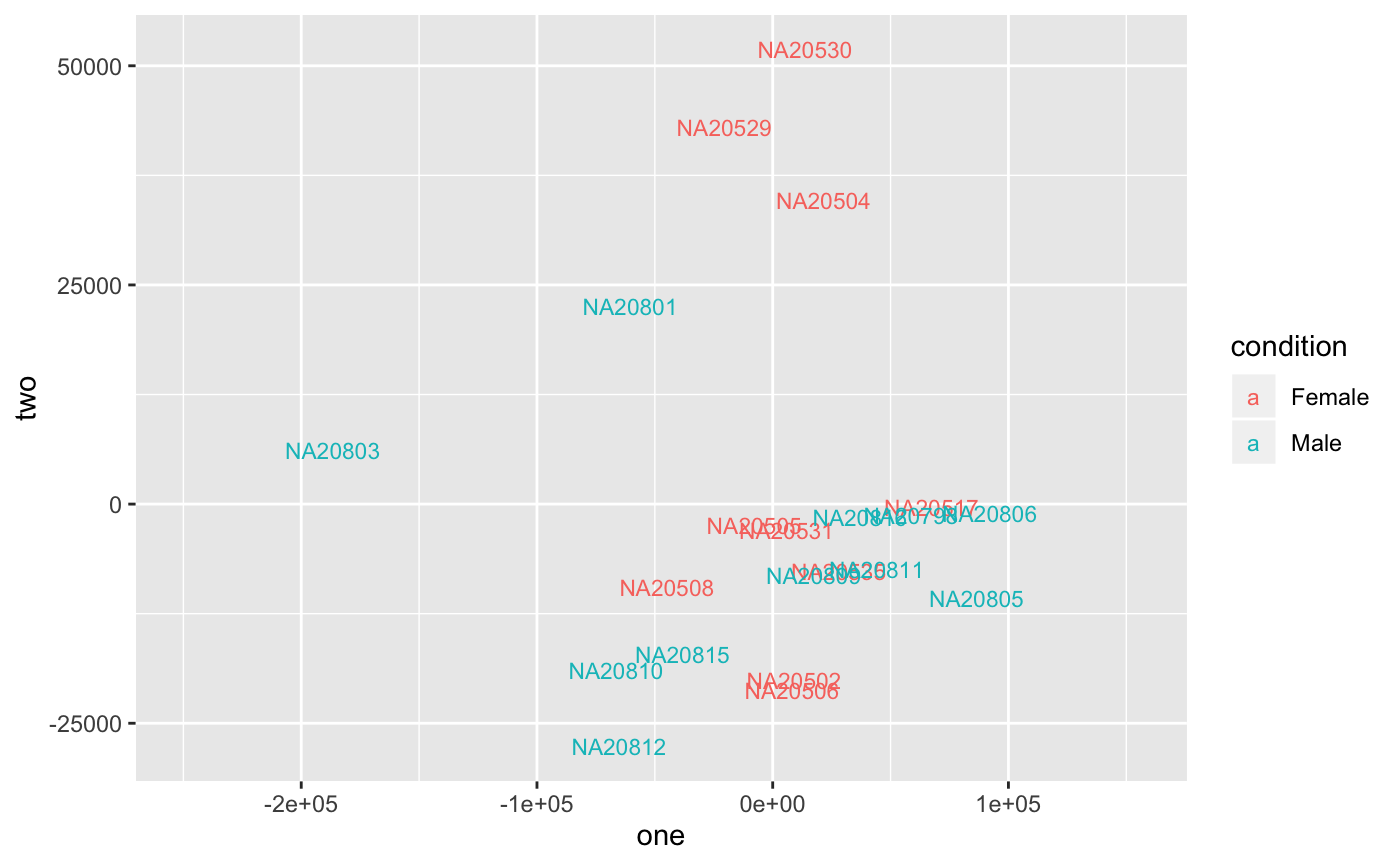Uses cmdscale to get multidimensional scaling of data matrix, and plot the samples with ggplot2.

degMDS(counts, condition = NULL, k = 2, d = "euclidian", xi = 1,
yi = 2)

## Arguments

counts matrix samples in columns, features in rows vector define groups of samples in counts. It has to be same order than the count matrix for columns. integer number of dimensions to get type of distance to use, c("euclidian", "cor"). number of component to plot in x-axis number of component to plot in y-axis

ggplot2 object

## Examples

data(humanGender)
library(DESeq2)
idx <- c(1:10, 75:85)
dse <- DESeqDataSetFromMatrix(assays(humanGender)[][1:1000, idx],
colData(humanGender)[idx,], design=~group)
degMDS(counts(dse), condition = colData(dse)[["group"]])